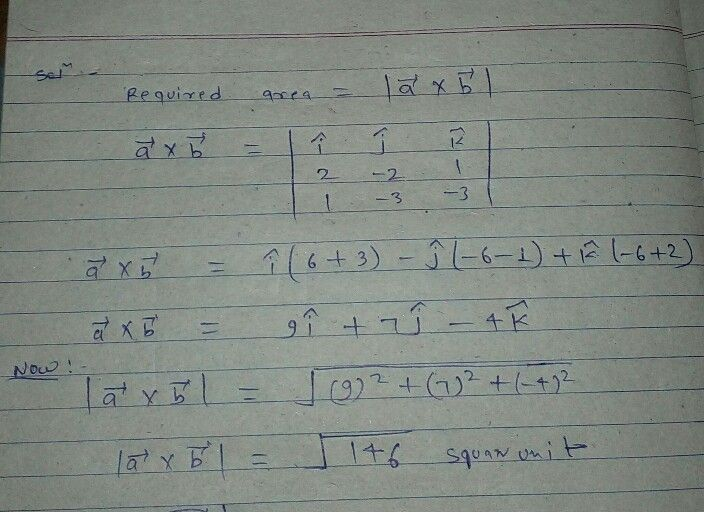Symbol
Problem$3$ Find the area of the parallelogram whose adjacent sides are the vectors $5=2i$ $=2\div k$ $2$ $5=i-3i=38$
10th-13th grade
Algebra
Search count: 167
SolutionQanda teacher - goru7